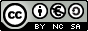Section: Geometry INDEX Introduction Aims Ratios in a unit circle: Relations between ratios of some angles:
TRIGONOMETRICAL RATIOS
INTRODUCTION

As Thales' theorem tells us, trigonometrical ratios of an angle do not depend on the right-angled triangle we choose. Therefore, we can choose a right-angled triangle whose hypotenuse is the radius of a unit circle.

We have drawn a set of axes and used the origin as the centre of an auxiliary circle whose radius is equal to one unit. We have drawn a right-angled triangle in the circle, which we will use to define the trigonometrical ratios. By choosing a radius of this length we have made it easier to find, for each trigonometrical ratio of a given angle, a segment whose length is equal to this ratio.

This circle whose radius is equal to one unit is called the unit circle.

AIMS
• To learn the trigonometrical ratios of angles.

• To find the relation between the different trigonometrical ratios of the same angle.

• To find the relation between the trigonometrical ratios of complementary and supplementary angles, as well as angles with a difference of p radians and opposite angles.

• Recognise the relation that exists between the sides of a right-angled triangle.

 Pedro Férez MartínezSpanish Ministry of Education. Year 2001